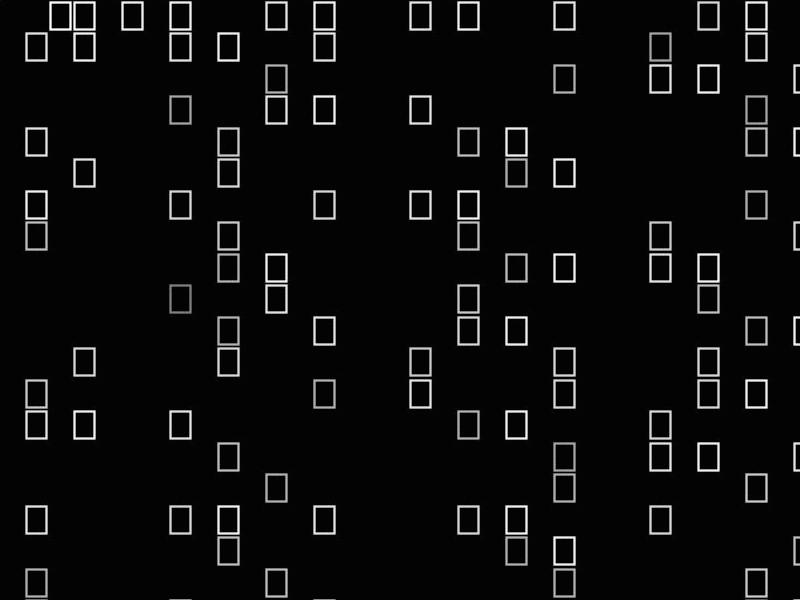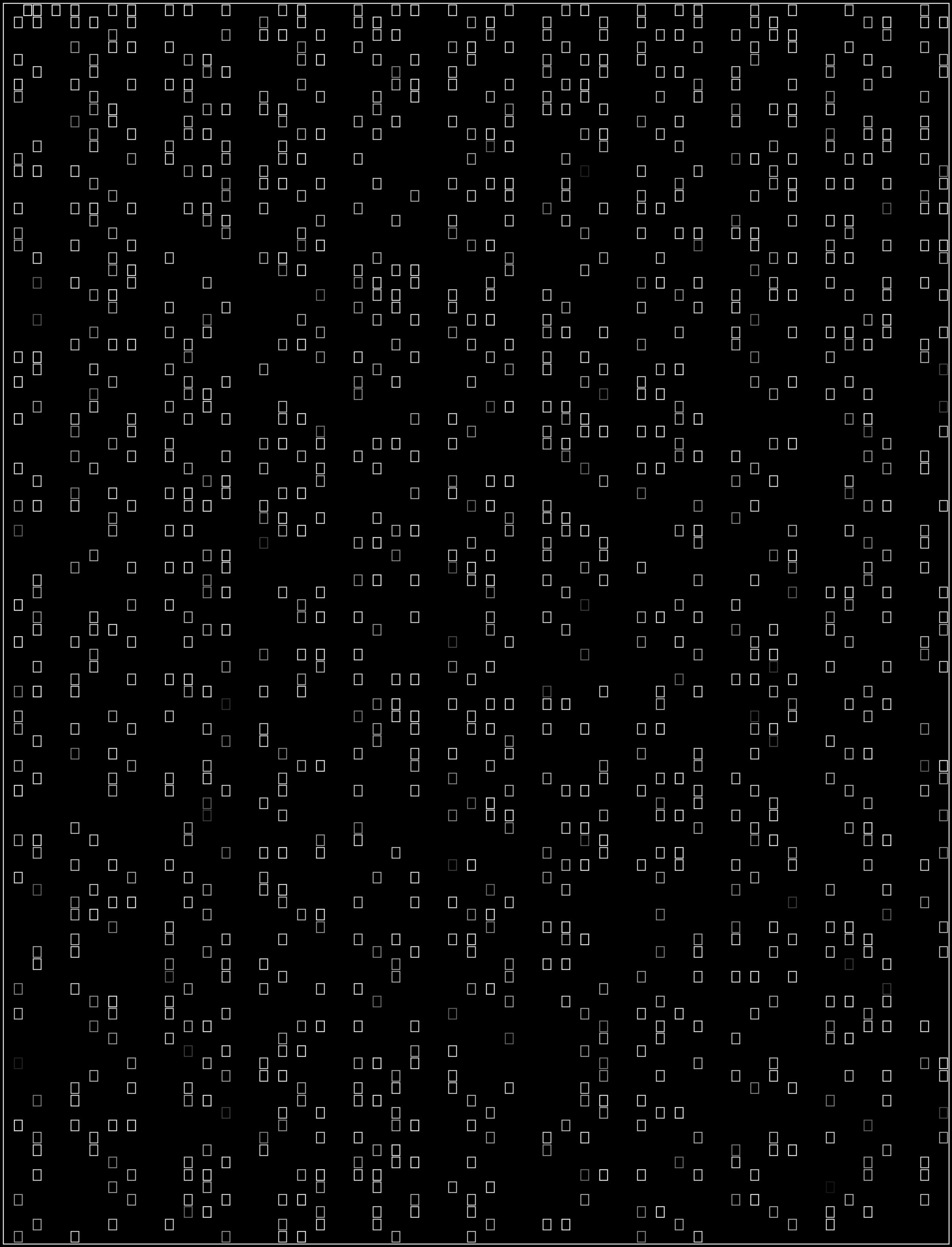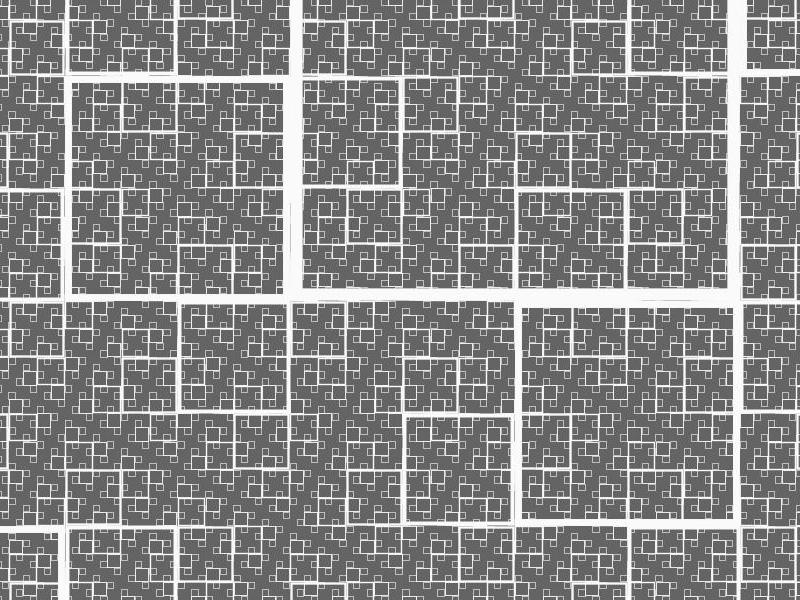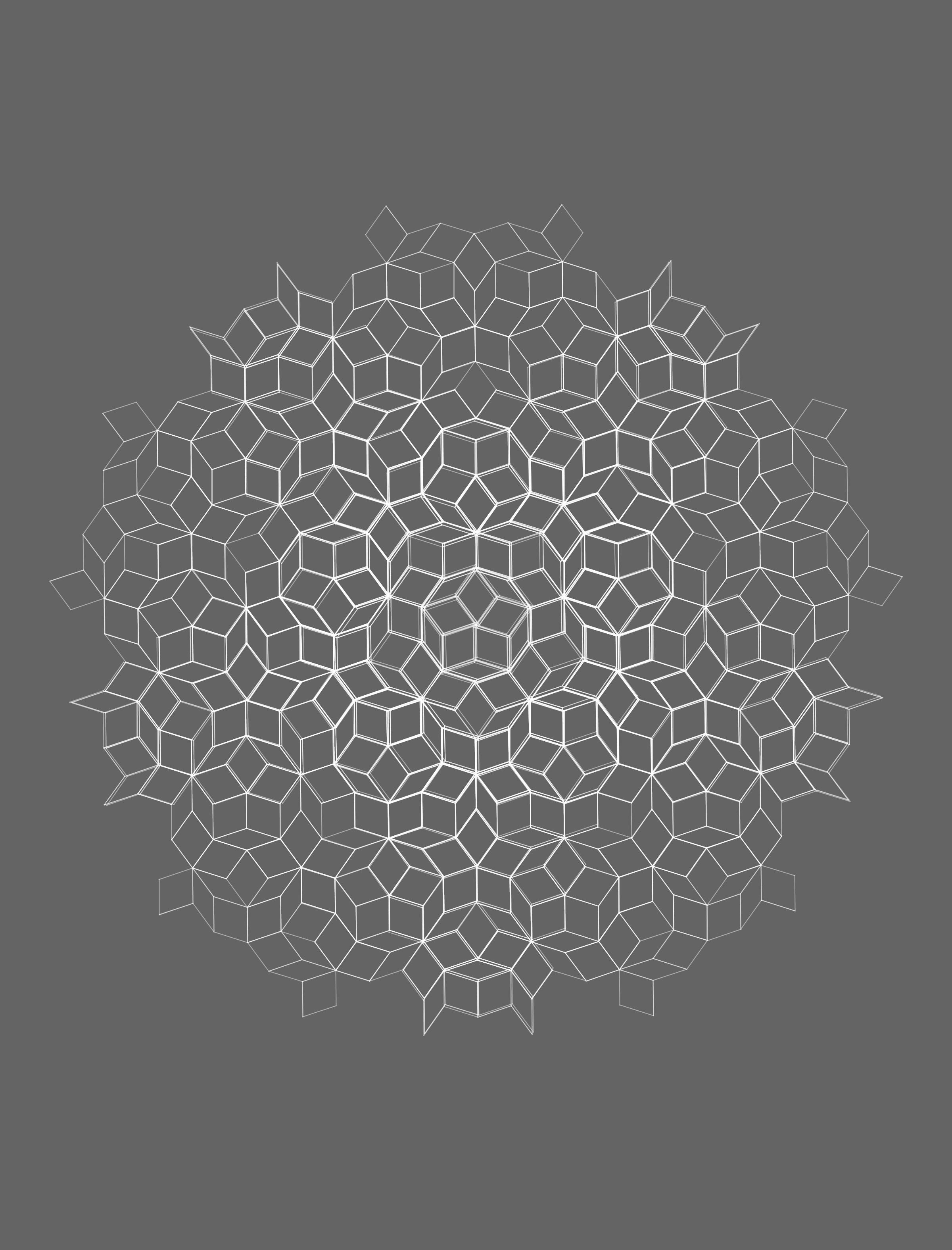Processing

A collection of Processing sketches that generate various artistic images.

• Erato Sieve - The first 10,000 prime numbers printed as boxes on a grid using the Sieve of Eratosthenes.
• Square Fractal - Cantor like fractals generated by some simple recursion.
• Penrose Tile - Penrose tiles generated using L system rules.

on github

• Processing
• Math + Art
• Fractals
•a visual plot of the first 10,000 prime numbers (zoomed in)

•a visual plot of the first 10,000 prime numbers

•noisy fractals

•noisy penrose tiles

Back to all projects